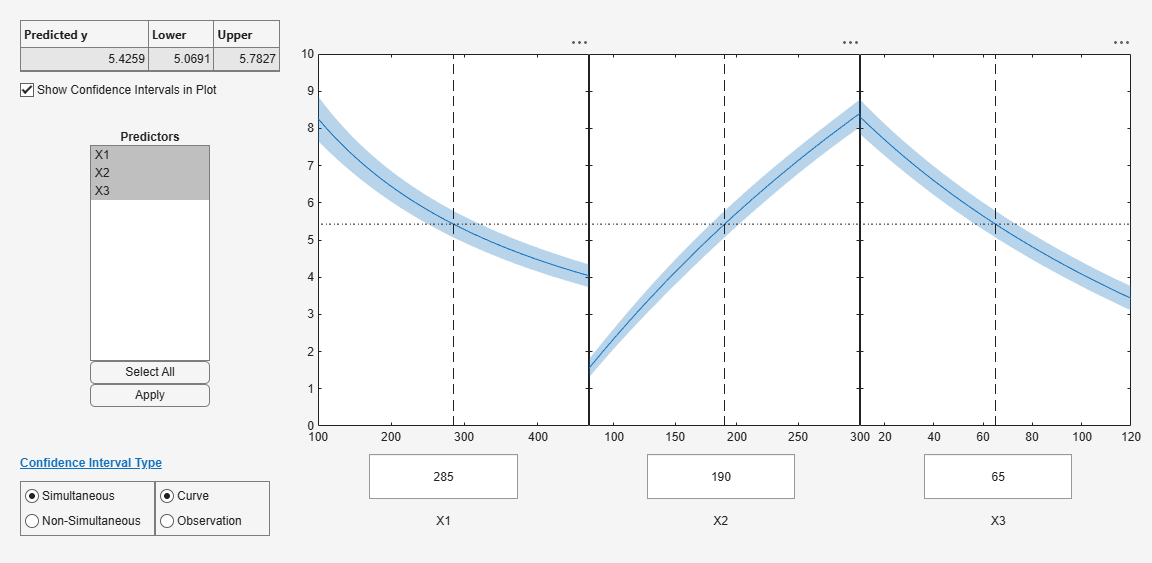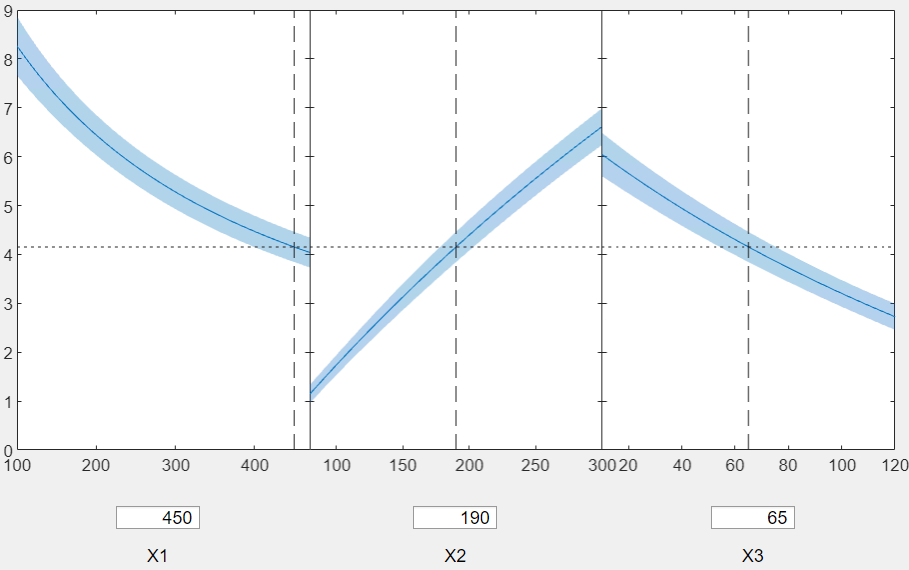# plotSlice

Plot of slices through fitted nonlinear regression surface

## Syntax

``plotSlice(mdl)``

## Description

example

````plotSlice(mdl)` creates a figure containing one or more plots, each representing a slice through the regression surface predicted by `mdl`. Each plot shows the fitted response values as a function of a single predictor variable, with the other predictor variables held constant.`plotSlice` also displays the 95% confidence bounds for the response values. Use the Bounds menu to choose the type of confidence bounds. Use the Predictors menu to select which predictors to use for each slice plot. For details, see Tips.```

## Examples

collapse all

Plot slices of a fitted nonlinear model.

Load the `reaction` data and fit a model of the reaction rate as a function of reactants.

```load reaction mdl = fitnlm(reactants,rate,@hougen,[1 .05 .02 .1 2]);```

Create a slice plot.

`plotSlice(mdl)`Drag the `X1` prediction line to the right, and observe the change in the predicted response `y` and in the predicted response curves to `X2` and `X3`.## Input Arguments

collapse all

Nonlinear regression model object, specified as a `NonLinearModel` object created by using `fitnlm`.

## Tips

• Use the Bounds menu in the figure window to choose the type of confidence bounds. You can choose Simultaneous or Non-Simultaneous, and Curve or Observation. You can also choose No Bounds to have no confidence bounds.

• Simultaneous or Non-Simultaneous

• Simultaneous (default) — `plotSlice` computes confidence bounds for the curve of the response values using Scheffe's method. The range between the upper and lower confidence bounds contains the curve consisting of true response values with 95% confidence.

• Non-Simultaneous — `plotSlice` computes confidence bounds for the response value at each observation. The confidence interval for a response value at a specific predictor value contains the true response value with 95% confidence.

Simultaneous bounds are wider than separate bounds, because requiring the entire curve of response values to be within the bounds is stricter than requiring the response value at a single predictor value to be within the bounds.

• Curve or Observation

A regression model for the predictor variables X and the response variable y has the form

y = f(X) + ε,

where f is a function of X and ε is a random noise term.

• Curve (default) — `plotSlice` predicts confidence bounds for the fitted responses f(X).

• Observation`plotSlice` predicts confidence bounds for the response observations y.

The bounds for y are wider than the bounds for f(X) because of the additional variability of the noise term.

• Use the Predictors menu in the figure window to select which predictors to use for each slice plot. If the regression model `mdl` includes more than eight predictors, `plotSlice` creates plots for the first five predictors by default.

## Version History

Introduced in R2012a